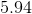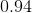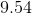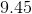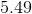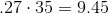# Algebra 1 : How to find decimal equivalent to a percentage

## Example Questions

### Example Question #21 : How To Find Decimal Equivalent To A Percentage

Find the decimal equivalent of the following percent: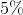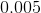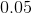Explanation:

In order to find the decimal equivalent for a percent, all we have to do is divide our percent by 100. We can also move the decimal point to the left two spaces.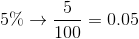### Example Question #22 : How To Find Decimal Equivalent To A Percentage

Find the decimal equivalent of the following percent: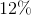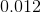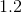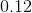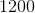Explanation:

In order to find the decimal equivalent for a percent, all we have to do is divide our percent by 100. We can also move the decimal point to the left two spaces.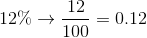### Example Question #23 : How To Find Decimal Equivalent To A Percentage

Find the decimal equivalent of the following percent: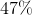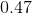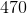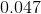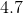Explanation:

In order to find the decimal equivalent for a percent, all we have to do is divide our percent by 100. We can also move the decimal point to the left two spaces.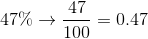### Example Question #21 : How To Find Decimal Equivalent To A Percentage

What is the decimal equivalen of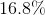?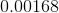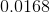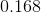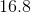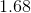Explanation:

To find the decimal equivalent of a percentage, take the percentage and divide it by one-hundred: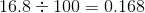### Example Question #25 : How To Find Decimal Equivalent To A Percentage

What is the decimal equivalent of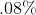?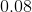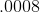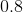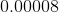Explanation:

When presented with a percentage, we can effectively "move" the decimal point over two spots and arrive at the equivalent decimal. In our example,turns toin decimal form.

### Example Question #26 : How To Find Decimal Equivalent To A Percentage

John makes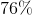of the shots he takes in a basketball game. What is this number written as a decimal?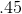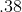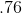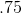Explanation:

To find a decimal from a percent, you just need to put the percent overSo: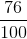This is equivalent to seventy-six hundreths which, as a decimal is written as.

### Example Question #27 : How To Find Decimal Equivalent To A Percentage

If you owe the bank% of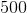dollars, how much money do you owe them?  Round your answer to the nearest cent.Explanation:

To solve this problem, first, you must convert the percentage above into a decimal.  To find the decimal equivalent of a percentage, simply move the decimal (if there is none, remember that there is always an implied decimal after the one's digit) to the left twice.  Then, you take your result and multiply it by the initial amount for your answer: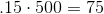### Example Question #28 : How To Find Decimal Equivalent To A Percentage

Which of the following is the decimal equivalent of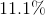?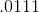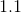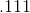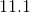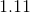Explanation:

To find the decimal equivalent of a percentage, simply move the decimal (if there is none, remember that there is always an implied decimal after the one's digit) to the left twice.  The best answer is:### Example Question #29 : How To Find Decimal Equivalent To A Percentage

Which of the following is the decimal equivalent of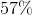?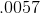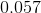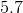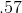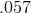Explanation:

To find the decimal equivalent of a percentage, simply move the decimal (if there is none, remember that there is always an implied decimal after the one's digit) to the left twice.  The best answer is:### Example Question #30 : How To Find Decimal Equivalent To A Percentage

If you owe the bank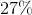ofdollars, how much money do you owe them?  Round your answer to the nearest cent.